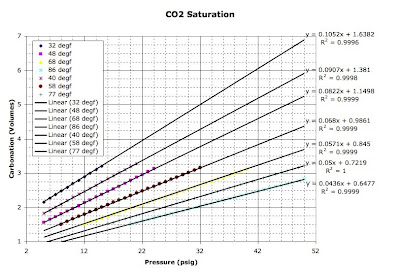Thursday, June 25, 2009

Multivariable Equations

When I was working as an engineer, I came up with a way to combine two physical dependencies into one equation (I'm sure a lot of people have figured the same thing out, but I'm still proud of myself). I think my original intent was to predict a jet engine's maximum thrust at a given temperature and pressure, but I don't remember for sure. Whatever it was, the method works beautifully for determining a beer's carbonation level at a given temperature and pressure. Here's how to do it:

-Plot the values from a carbonation table (I used an American Society of Brewing Chemists version) and create linear equations of CO2 volumes vs. pressure at each temperature. It should look like this, but without the haphazard legend order:-Each equation has a first order coefficient and a constant. We'll call those values x1 and x0 respectively. Note that CO2 volumes = x1*P + x0 at a given temperature.
-Plot the x1 values vs. temperature and create an equation that describes the relationship:-Do the same thing with the x0 values:-Replace the coefficients of the pressure equation with the equations that describe x1 and x0. The result is this:

CO2 volumes = x1*P + x0 = ((0.00000981*T-0.00229169)*T+0.16809729)*P+(0.00024826*T-0.04710192)*T+2.87424296

You could just look up CO2 volumes in a table instead, but what fun is that?

1 comment:

Gage Mitchell said...

Wow. Beer geek meets engineering nerd. Very nice. I like it.

Regards,
Gage